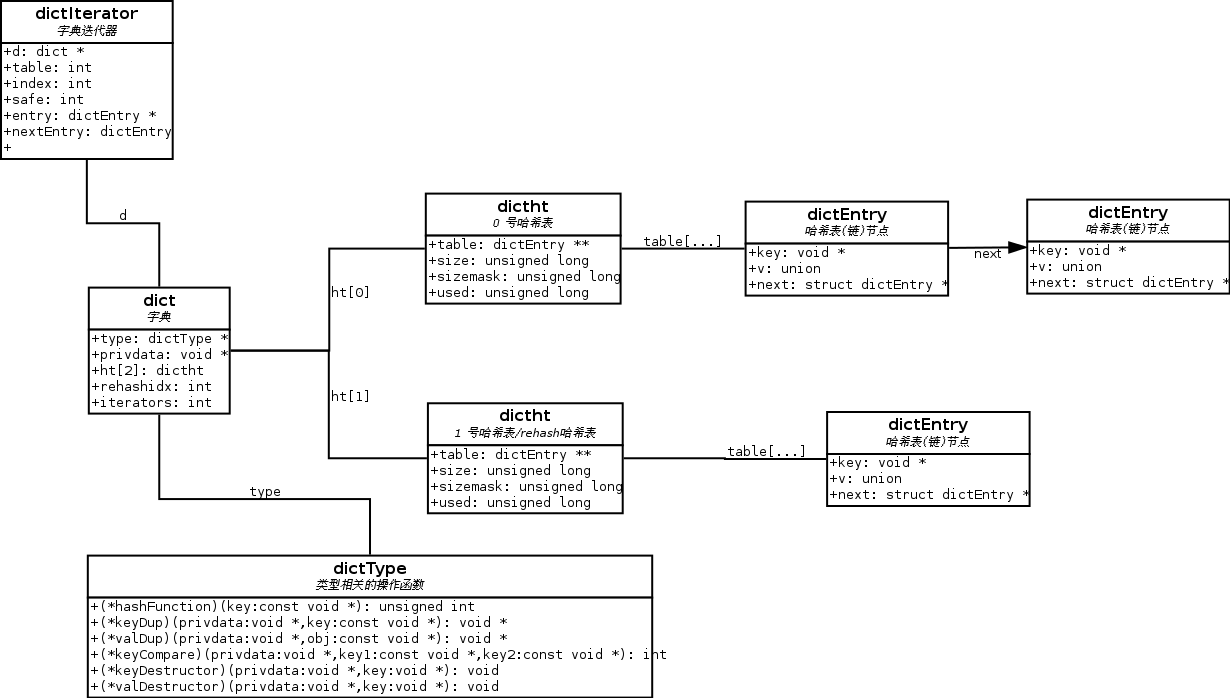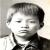# Redis 源码分析：dict.c 和 dict.h

### 数据结构概览

dict.h 中定义了被 dict.c 的程序所使用的几个数据结构，如 dict 、dictht 和 dictEntry 等，它们之间的关系可以用下图来描述(点击放大）：### 数据结构实现细节

dict 结构的定义如下：

```/* 字典结构 */
typedef struct dict {
dictType *type;     // 为哈希表中不同类型的值所使用的一族函数
void *privdata;
dictht ht;       // 每个字典使用两个哈希表
int rehashidx;      // 指示 rehash 是否正在进行，如果不是则为 -1
int iterators;      // 当前正在使用的 iterator 的数量
} dict;```

```typedef struct dictht {
dictEntry **table;      // 节点指针数组
unsigned long size;     // 桶的数量
unsigned long used;     // 已有节点数量
} dictht;```

table 属性组成了一个数组，数组里带有节点指针，用作链表。

size 、 sizemask 和 used 这三个属性初看上去让人有点头晕，实际上，它们分别代表的是：

size ：桶的数量，也即是， table 数组的大小。

sizemask ：这个值通过 size – 1 计算出来，给定 key 的哈希值计算出来之后，就会和这个数值进行 & 操作，决定元素被放到 table 数组的那一个位置上。

used ：这个值代表目前哈希表中元素的数量，也即是哈希表总共保存了多少 dictEntry 结构。

```typedef struct dictEntry {
void *key;              // 键
union {
void *val;
uint64_t u64;
int64_t s64;
} v;                    // 值(可以有几种不同类型)
struct dictEntry *next; // 指向下一个哈希节点(形成链表)
} dictEntry;```

### 字典创建流程

OK，说回来字典这边，创建新字典执行的调用链是： dictCreate -> _dictInit -> _dictReset

```static void _dictReset(dictht *ht)
{
ht->table = NULL;
ht->size = 0;
ht->used = 0;
}```

### 0 号哈希表的创建流程

```dictEntry *dictAddRaw(dict *d, void *key)
{
// 被省略的代码...

// 计算 key 的 index 值
// 如果 key 已经存在，_dictKeyIndex 返回 -1
if ((index = _dictKeyIndex(d, key)) == -1)
return NULL;

// 被省略的代码...
}```

```static int _dictKeyIndex(dict *d, const void *key)
{
// 被省略的代码...

/* Expand the hashtable if needed */
if (_dictExpandIfNeeded(d) == DICT_ERR)
return -1;

// 被省略的代码...
}```

```static int _dictExpandIfNeeded(dict *d)
{
// 被忽略的代码...

/* If the hash table is empty expand it to the intial size. */
if (d->ht.size == 0) return dictExpand(d, DICT_HT_INITIAL_SIZE);

// 被忽略的代码...
}```

dictExpand 会创建一个分配了链表数组的新哈希表，然后进行判断，决定是该将新哈希表赋值给 0 号哈希表还是 1 号哈希表。

```int dictExpand(dict *d, unsigned long size)
{
// 被省略的代码...

// 计算哈希表的(真正)大小
unsigned long realsize = _dictNextPower(size);

// 创建新哈希表
dictht n;
n.size = realsize;
n.table = zcalloc(realsize*sizeof(dictEntry*));  // 分配链表数组
n.used = 0;

// 字典的 0 号哈希表是否已经初始化？
// 如果没有的话，我们将新建哈希表作为字典的 0 号哈希表
if (d->ht.table == NULL) {
d->ht = n;
} else {
// 否则，将新建哈希表作为字典的 1 号哈希表，并将它用于 rehash
d->ht = n;
d->rehashidx = 0;
}

// 被省略的代码...
}```

### 字典的扩展和 1 号哈希表的创建

（字典的）哈希表的完整 rehash 操作分为两步：

1） 首先就是创建一个比现有哈希表更大的新哈希表（expand）

2） 然后将旧哈希表的所有元素都迁移到新哈希表去（rehash）

```static int _dictExpandIfNeeded(dict *d)
{
// 被省略的代码...

// expand 有两种
// 1) used >= size 且 dict_can_resize 为真，正常 expand
// 2) dict_can_resize 不为真，但 used/size 大于指定比率，强制 expand
if (d->ht.used >= d->ht.size &&
(dict_can_resize ||
d->ht.used/d->ht.size > dict_force_resize_ratio))
{
return dictExpand(d, ((d->ht.size > d->ht.used) ?
d->ht.size : d->ht.used)*2);
}

// 被省略的代码...
}```

```int dictExpand(dict *d, unsigned long size)
{
// 被省略的代码...

// 计算哈希表的(真正)大小
unsigned long realsize = _dictNextPower(size);

// 创建新哈希表
dictht n;
n.size = realsize;
n.table = zcalloc(realsize*sizeof(dictEntry*));
n.used = 0;

// 字典的 0 号哈希表是否已经初始化？
// 如果没有的话，我们将新建哈希表作为字典的 0 号哈希表
if (d->ht.table == NULL) {
d->ht = n;
} else {
// 否则，将新建哈希表作为字典的 1 号哈希表，并将它用于 rehash
d->ht = n;
d->rehashidx = 0;
}

// 被省略的代码...
}```

### 渐增式 rehash 和平摊操作

```dictEntry *dictFind(dict *d, const void *key)
{
// 被忽略的代码...

// 检查字典(的哈希表)能否执行 rehash 操作
// 如果可以的话，执行平摊 rehash 操作
if (dictIsRehashing(d)) _dictRehashStep(d);

// 被忽略的代码...
}```

`#define dictIsRehashing(ht) ((ht)->rehashidx != -1)`

```static void _dictRehashStep(dict *d) {
if (d->iterators == 0) dictRehash(d,1);
}```

（代码中的 iterators == 0 表示在 rehash 时不能有迭代器，因为迭代器可能会修改元素，所以不能在有迭代器的情况下进行 rehash 。）

```int dictRehash(dict *d, int n) {
// 被忽略的代码...

while(n--) {
dictEntry *de, *nextde;

// 0 号哈希表的所有元素 rehash 完毕？
if (d->ht.used == 0) {
zfree(d->ht.table);  // 清空 0 号
d->ht = d->ht;   // 用原来的 1 号代替 0 号

_dictReset(&d->ht);  // 重置 1 号哈希表
d->rehashidx = -1;      // 重置字典的 rehash flag

return 0;
}
// 被忽略的代码...
}

// 被忽略的代码...
}```

```dictEntry *dictAddRaw(dict *d, void *key)
{
// 被省略的代码...

// 如果字典正在进行 rehash ，那么将新元素添加到 1 号哈希表，
// 否则，使用 0 号哈希表
ht = dictIsRehashing(d) ? &d->ht : &d->ht;

// 被省略的代码...
}```

### 最后：哈希表的大小

```static int _dictExpandIfNeeded(dict *d)
{
// 被省略的代码...

if (d->ht.used >= d->ht.size &&
(dict_can_resize ||
d->ht.used/d->ht.size > dict_force_resize_ratio))
{
return dictExpand(d, ((d->ht.size > d->ht.used) ?
d->ht.size : d->ht.used)*2);
}

// 被省略的代码...
}```

```int dictExpand(dict *d, unsigned long size)
{
// 被省略的代码...

// 计算哈希表的(真正)大小
unsigned long realsize = _dictNextPower(size);

// 创建新哈希表
dictht n;
n.size = realsize;
n.table = zcalloc(realsize*sizeof(dictEntry*));
n.used = 0;

// 被省略的代码...
}```

```static unsigned long _dictNextPower(unsigned long size)
{
unsigned long i = DICT_HT_INITIAL_SIZE;

if (size >= LONG_MAX) return LONG_MAX;
while(1) {
if (i >= size)
return i;
i *= 2;
}
}```

1） 哈希表的大小总是 2 的乘幂（也即是 2^N，此处 N 未知）

2）1 号哈希表的大小总比 0 号哈希表大

### 就这样了！

dict 的结构本身还是很简单的，唯一的遗憾是，dictht 作为字典的内部实现，本该被隐藏起来的，现在却完全暴露了出来（什么， _dictReset(&d->ht)，你是说真的？），如果能在模块化方面多做一点努力的话，代码会更容易看一些，当然这里也有历 史遗留的因素在里面。

00
i